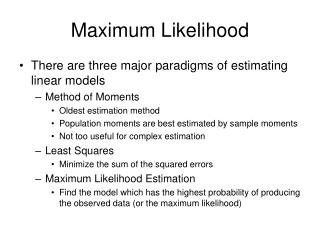DownloadDownload PresentationMaximum Likelihood

# Maximum Likelihood

Download Presentation## Maximum Likelihood

- - - - - - - - - - - - - - - - - - - - - - - - - - - E N D - - - - - - - - - - - - - - - - - - - - - - - - - - -
##### Presentation Transcript

1. Maximum Likelihood • There are three major paradigms of estimating linear models • Method of Moments • Oldest estimation method • Population moments are best estimated by sample moments • Not too useful for complex estimation • Least Squares • Minimize the sum of the squared errors • Maximum Likelihood Estimation • Find the model which has the highest probability of producing the observed data (or the maximum likelihood)

2. MLE - A Simple Idea • Maximum Likelihood Estimation (MLE) is a relatively simple idea. • Different populations generate different samples, and any given sample is more likely to have come from one population versus some other

3. An Illustration • Suppose you have 3 different normally distributed populations, • And a set of data points, x1, x2, …, x10

4. Parameters of the Model • Given that they are normally distributed, they differ only in their mean and standard deviation • The population with a mean of 5 will generate sample with a mean close to 5 more often than populations with a mean closer to 6 or 4.

5. More likely … • It is more likely that the population with the mean of 5 generate the sample than one (or any other) of the populations • Variances can factor into this likelihood as well.

6. A Definition of MLE • If a random variable X has a probability distribution f(x) characterized by parameters θ1, θ2, .. θk and if we observe a sample x1, x2, .. xn then the maximum likelihood estimators ofθ1, θ2, .. θk are those values of these parameters that would generate the sample most often

7. An example • Suppose X is a binary variable that can take on the value of 1 with probability of π f(0) = 1 – π f(1) = π • Suppose a random sample from this population is drawn: {1, 1, 0}

8. The MLE of π • Let us consider values for π between 0.0 and 1.0 • If π = 0.0, there are no successes and we could not generate the sample. (Similarly, 1.0 won’t work either – we couldn’t observe the 0. • But what about π = .1

9. π = .1 • The probability of drawing our sample would be estimated as: f(1, 1, 0) = f(1)f(1)f(0) = .1 x .1 x .9 = .009 • Because the joint probability of independent events is equal to the product of the simple events

10. A Grid search for π

11. Our MLE of π • Give the iterative grid search, we would conclude that our MLE for π would equal .7 • Yes, if we took it to the next significant digit, it would be .67. • Hence we would say that a population with π = .7 would be more likely to generate sample of {1, 1, 0} more often than any other population

12. The Likelihood Function • In order to derive MLEs we therefore need to express the likelihood function l. l = f(x1, x2, … xn) • And if the observations are independent: l = f(x1)f(x2) … f(xn)

13. To find MLE • Like least squares, set the first derivative= 0.0 • Also second derivative needs to be positive

14. Log-Likelihood • For some reason, the log-likelihood is easier to find. • The logs of multiplicative components are added, and some will therefore drop out, making derivatives easier to estimate if a = bc log(a) = log(b) + log(c) • In addition, logs make otherwise intractably small numbers usable • (e.g.) Log10 .0000001 = -7.0 • This means that to maximize the likelihood, we need to minimize the negative of the log-likelihood.

15. Goodness-of-fit • -2 LLR is Chi-square with #parameters -1 degrees of freedom

16. MLE - Definitions • The MLEs of the parameters of a given population are those values which will generate the observed sample most often • Find likelihood function • Maximize it • Indicate goodness-of-fit and inference • Inference is based on the assumption of normality, and thus the test statistics are z statistics